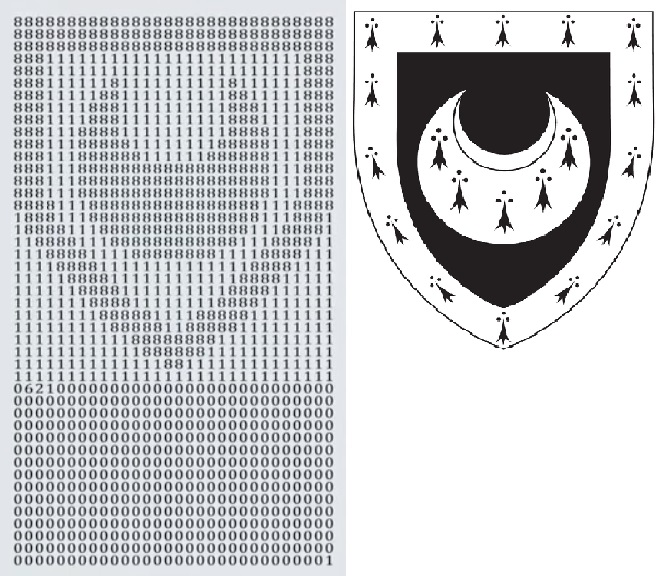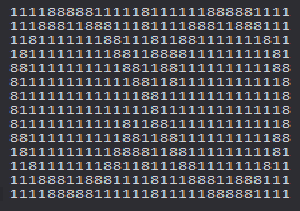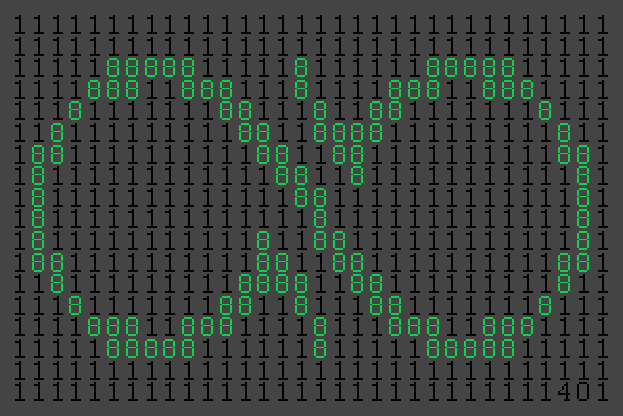# The Trinity Hall Prime and Other Pixel Primes

About 3 months ago I watched this video by Numberphile on the "Trinity Hall Prime". You can watch the video but here's a quick summary. As a graduation gift, a mathematician from Trinity Hall produced the following 1350-digit prime number, and printed it out to be framed. The top bit is the crest of the school composed out of 1's and 8's, followed by a (nearly) unmodified series of 0's, and finally ends with a single 1.The reason it's 1350 digits long is to commemorate the year that Trinity Hall was founded. However, you'll notice that right at the beginning of the 0's there's an anomality: it starts with 0621. It's obviously unlikely that any given number composes solely of 8's, 1's, and 0's would be prime especially one with a pattern. This anomaly is where the number can be randomly modified until a prime number is found.

However, this makes it relatively straight forward. This process can be relatively easily replicated using Mathematica and some code. For demonstration purposes I'll choose a more simple method of modifying the prime. First, take the image that you want to use. I'll use my logo.Then take it into Photoshop, convert it to two colors using Image > Adjustments > Threshold, and then resize the canvas down to the dimensions that you want. I'll do 30 pixels wide (the Trinity Hall Prime is 29), which gives me a height of 14 pixels. Threshold again to assure you're at only 2 colors, white and black, and begin to copy that into 1's and 8's. I just did this by hand. Here's a slightly distorted version of the straight numbers to show the image:If you squint you can MAYBE make it out. Here's the number without any formatting: 111188888111118111111888881111111888118881118111188811888111118111111188111811881111111811181111111118811888811111111181881111111111881188111111111188811111111111188118111111111118811111111111118811111111111118811111111111111811111111111118811111111111811881111111111118881111111111881188111111111188181111111118888118811111111181118111111188118111881111111811111888118881111811188811888111111188888111111811111888881111.

I'm going to add a full border of 1's to the image to delineate even further. Then for simplicity's sake, I'll just accept that the anomaly can be at the very end, and use the `NextPrime` command in Mathematica to generate the next prime number from that long number above. Mathematica tells me that the next prime in question ends with 401 instead of 111, but is otherwise unchanged. With some formatting, here's my unique "Alex Beals Prime"!This is relatively straight forward to do with any image that can easily be converted into two colors. Doing a specific number of digits is also relatively straight forward as long as it factors well. As an example, Dartmouth was founded in 1769. 1769 factors into 29 x 61, so when you resize your image just make sure it's 29 pixels wide, and then pad the top and bottom with lines of 1's until it's 61 rows long: easy!

In case you're curious, here's the raw number: 111111111111111111111111111111111111111111111111111111111111111111111888881111181111118888811111111188811888111811118881188811111118111111188111811881111111811111811111111188118888111111111811188111111111188118811111111118811811111111111188118111111111118118111111111111188111111111111181181111111111111181111111111111811811111111111811881111111111118118811111111118811881111111111881118111111111888811881111111118111118111111188118111881111111811111118881188811118111888118881111111118888811111181111188888111111111111111111111111111111111111111111111111111111111111111111401, which is 576 digits long!